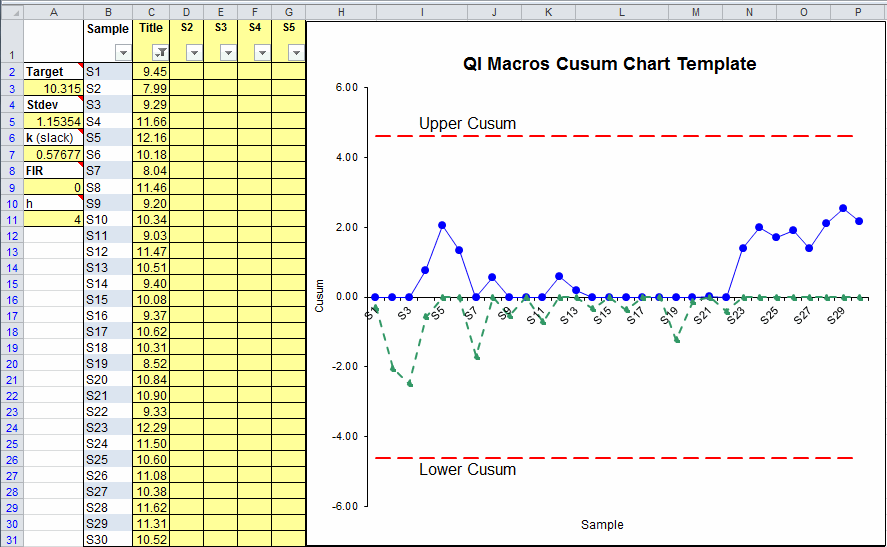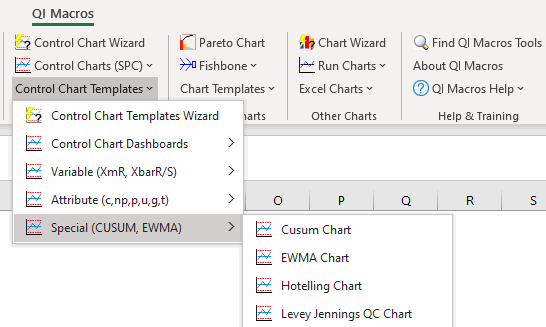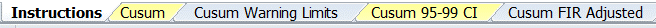# Looking for a Cusum Chart Template in Excel?### Cumulative Sum Charts are Used to Detect Small Shifts in a Process

The Cusum Chart can detect process shifts more rapidly than the Individuals Moving Range (XmR) or XbarR Charts. If a trend develops, it's evidence that the process has shifted.

### A Cusum Chart consists of the following:

• A target which is either provided by you or calculated as the mean of your data.
• Upper Cusum Limit and Lower Control Limit lines.
• C+ and C- lines which represent the cumulative sum of deviations of successive sample means from a target. Use these lines to determine if your process is shifting.

### Cusum Chart Template in QI Macros for Excel:

QI Macros add-in for Excel contains an easy to use Cusum Chart template. The template is pre-populated with sample data to show you how the template works. Just delete the sample data or type your data over the filled in cells.

### To Create a Cusum Chart:

1. Input your Target value in cell A3.
2. Input labels in column B and chart title in cell C1.
3. Input data into columns C to G. Just use column C if your subgroup size is one. As you input data in columns C to G, the chart will populate to the right.
4. St Dev in cell A5 and k (slack) in cell A7 will also be calculated. k (slack) defines the amount of shift from the target you want to be able to detect. This is an estimated value, calculated at 1/2 of StDev, but can be changed.
5. Fast Initial Response (FIR) value defaults to 0. This feature can be used to set an initial "head start" value to improve detection of an out-of-control condition at the start of the chart. If the process is in control, this initial head start has little effect; if the process is out of control, a signal will be given faster with a non-zero value than with a zero value.
6. h is called the threshold value or decision interval. It determines how many StDev from the mean the Cusum Limits are set.

NOTE: Using h=4 or h=5 and k=.5 will generally provide a cusum that has good ARL properties (per Montgomery SPC 4th ed.)

Find the Cusum Chart template by clicking on the QI Macros menu > Control Chart Templates > Special (CUSUM, EWMA) > Cusum Chart:### Cusum Template Options in QI Macros• Cusum: Standard template and formulas.
• Cusum Warning Limits: Adds warning limit lines to chart (like 2 sigma lines on a control chart).
• Cusum 95-99 CI: Uses confidence intervals to calculate warning limits.